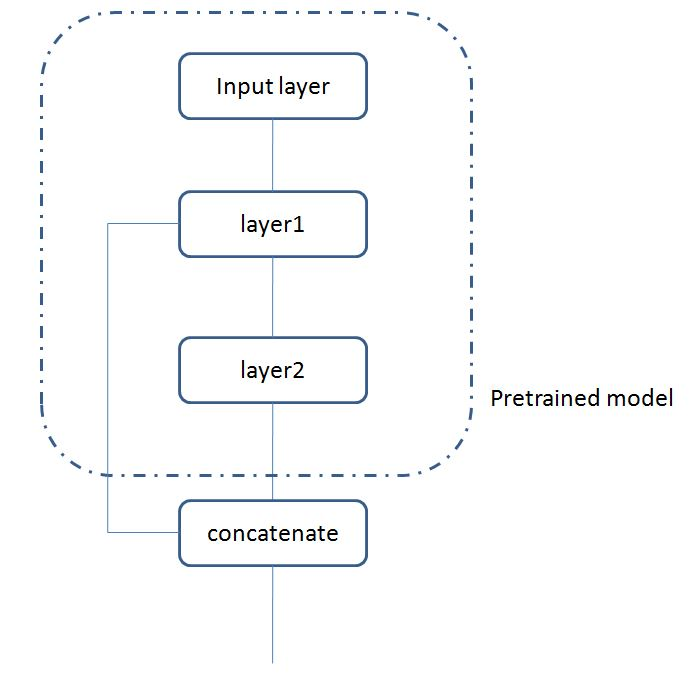``````    base_model = VGGFace(model='resnet50', include_top=False)
model1 = base_model
model2 = base_model

input1 = Input(shape=(197,197,3))
model1_out = model1.layers[-12].output
model1_in = model1.layers.output
model1 = Model(model1_in,model1_out)
x1 = model1(input1)
x1 = GlobalMaxPool2D()(x1)

x2 = model2(input1)
x2 = GlobalMaxPool2D()(x2)
out = Concatenate(axis=-1)([x1,x2])
out = Dense(1,activation='sigmoid')(out)
model3 = Model([input1,input2],out)

from keras.utils import plot_model
plot_model(model3,"model3.png")
import matplotlib.pyplot as plt
plt.imshow(img)

``````

1个回答

https://www.jianshu.com/p/ee2a170b3a12keras如何为已经训练好的模型添加层？

keras中使用预训练的VGG19网络能否处理单通道的灰度图？

keras搭的模型，训练时候，使用相同的loss和metrics，但是输出却不一样，为什么会出现这种情况呀

Keras能否实现GRNN模型，如果可以那程序是怎么样的？

```python batch_size = 128 original_dim = 100 #25*4 latent_dim = 16 # z的维度 intermediate_dim = 256 # 中间层的维度 nb_epoch = 50 # 训练轮数 epsilon_std = 1.0 # 重参数 #my tips:encoding x = Input(batch_shape=(batch_size,original_dim)) h = Dense(intermediate_dim, activation='relu')(x) z_mean = Dense(latent_dim)(h) # mu z_log_var = Dense(latent_dim)(h) # sigma #my tips:Gauss sampling,sample Z def sampling(args): # 重采样 z_mean, z_log_var = args epsilon = K.random_normal(shape=(128, 16), mean=0., stddev=1.0) return z_mean + K.exp(z_log_var / 2) * epsilon # note that "output_shape" isn't necessary with the TensorFlow backend # my tips:get sample z(encoded) z = Lambda(sampling, output_shape=(latent_dim,))([z_mean, z_log_var]) # we instantiate these layers separately so as to reuse them later decoder_h = Dense(intermediate_dim, activation='relu') # 中间层 decoder_mean = Dense(original_dim, activation='sigmoid') # 输出层 h_decoded = decoder_h(z) x_decoded_mean = decoder_mean(h_decoded) #my tips:loss(restruct X)+KL def vae_loss(x, x_decoded_mean): xent_loss = original_dim * objectives.binary_crossentropy(x, x_decoded_mean) kl_loss = - 0.5 * K.mean(1 + z_log_var - K.square(z_mean) - K.exp(z_log_var), axis=-1) return xent_loss + kl_loss vae = Model(x, x_decoded_mean) vae.compile(optimizer='rmsprop', loss=vae_loss) vae.fit(x_train, x_train, shuffle=True, epochs=nb_epoch, verbose=2, batch_size=batch_size, validation_data=(x_valid, x_valid)) vae.save(path+'//VAE.h5') ``` 一段搭建VAE结构的代码，在保存模型后调用时先是出现了sampling中一些全局变量未定义的问题，将变量改为确定数字后又出现了vae_loss函数未定义的问题（unknown loss function: vae_loss) 个人认为是模型中自定义的函数在保存上出现问题，但是也不知道怎么解决。刚刚上手keras和tensorflow这些框架，很多问题是第一次遇到，麻烦大神们帮帮忙！感谢！

Keras实现LSTM进行情感分析的问题，如何正确增加隐层

tensorflow 中怎么查看训练好的模型的参数呢？

keras实现人脸识别，训练失败……请教大神指点迷津！！！

keras模型的预测(predict)结果全是0

MySQL数据库面试题（2020最新版）

2020阿里全球数学大赛：3万名高手、4道题、2天2夜未交卷

Python爬虫，高清美图我全都要（彼岸桌面壁纸）

Java岗开发3年，公司临时抽查算法，离职后这几题我记一辈子